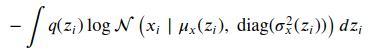# How to manually obtain the minus log-likelihood?

I’m implementing a VAE and I want to obtain the minus log-likelihood manually (not using an existing function). The given equation is. Another equation for it (the one implemented below) is available at Log-likelihood (couldn’t attach another picture). Been stuck in this for a couple of days now, don’t know where my code is wrong.

``````def loss_loglik(y_mean, y_logvar, x):
out_1 = (x.size()*x.size() / 2) * np.log(2 * np.pi)
out_2 = (x.size()*x.size() / 2) * torch.log(y_logvar.exp())
x_diff = x - y_mean
out_3 = torch.sum(x_diff.pow(2)) / (2 * y_logvar.exp())
loss = out_1 + out_2 + out_3

``````

The shape of the three arguments is (batch_size, 1, 28, 28).